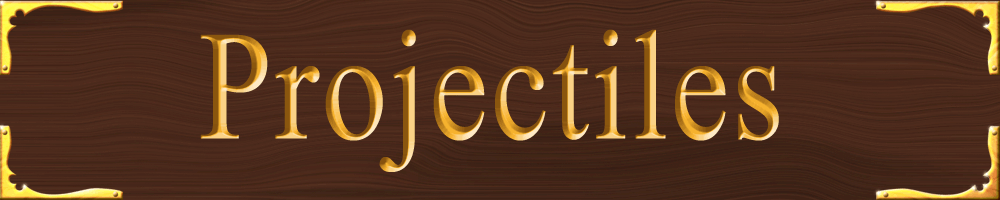## Free Fall

Free fall problems can often be solved by using:
vf = vi + at
to find v or t
d = vit + ½at2
to find d or t
or
vf2 = vi2 +2ad to find v or d

However, the vi portion of the equation will turn into zero and can be crossed out, if it drops from rest (vi = 0). Mathematically, it will also work out the same way (crossing out the vi portion) if the object is thrown upwards and reaches the peak (where its velocity will = 0)

### Free Fall Problem:

You have been sent to Pluto by NASA to fix a 90-meter high radio antenna. The antenna must function in order for NASA to communicate with astronauts sent to explore the planet Xena and its moon Gabrielle. While working on the antenna, you drop your wrench. It takes the wrench 21.2 seconds to fall to the cold surface of the Dwarf Planet.
1. Calculate the acceleration due to gravity on Pluto.

2. If you jump up at 5.4 m/s, how long will you stay in the air (round trip)?

3. Could you jump back to the top of the antenna if you jump at 5.4m/s?

 1. take inventory: d = -90m (negative because the direction is down) vi = 0m/s t = 21.2s ag = ? select equation: d = vit + 1⁄2at2 substitute and solve: -90m = 0m/s + (.5)(a)(449.44s2) a = -.4m/s2 (negative because gravity pulls down) 2. take inventory (slightly different parameters) vi = 5.4m/s vf = -5.4m/s (negative because the velocity is pointed down) ag = -.4m/s2 t = ? select equation: vf = vi + at substitute and solve: -5.4m/s = 5.4m/s + (-.4m/s2) (t) t = 27s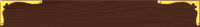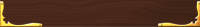## Worksheets

Compatability Pack for converting docx files to doc files

Free Fall Problems 1

Horizontally Launched Projectiles 1

Horizontally Launched Projectiles 2

Horizontally Launched Projectiles 3

Projectiles at an Angle Worksheet 13. This question has vi = 5.4m/s but the vf = 0m/s because when it reaches the peak the velocity is zero. Also, and this is very important, the time here is half of the round trip. Obvious but often overlooked. inventory: vi = 5.4m/s vf = 0m/s ag = -.4m/s2 d = ? (how does this distance compare to 90m?) select equation: vf2 = vi2 + 2ad substitute and solve: 0m2/ss2 = 29.6m2/s2 + (2)(-.4m/s2)(d) -29m2/s2 = -.8m/s2(d) 36.25m = d No. You cannot jump back up.Here's a video of climbing a tower. I love this one. It gives me the willies down in my gut.

## Horizontal Projectiles

### Consider this:

A ball rolling across a frictionless surface, with no air resistance, at 2m/s will continue rolling at 2m/s forever.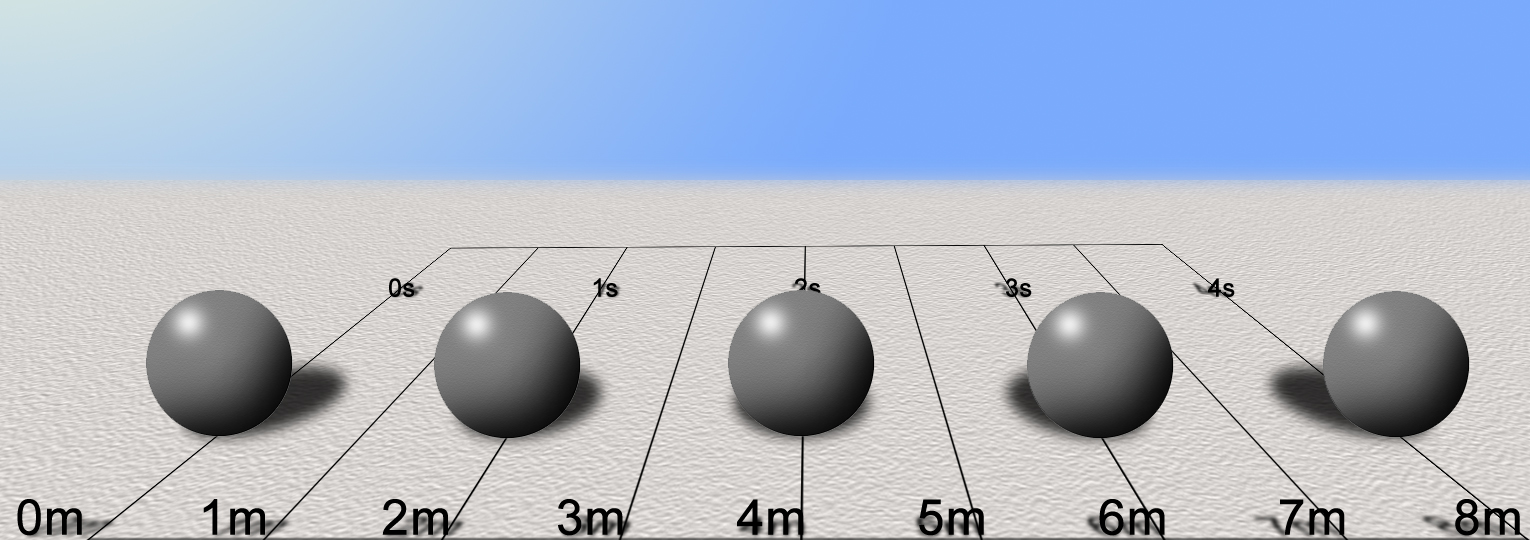In space, with no air resistance and no surface to rest on, the ball will go in a straight line at the same speed forever.

Now plot this on a graph:

Set up a graph in portrait orientation (up & down “hot dog”). Place the x-axis at the top and number it 0 through 70 as shown below. The label the y-axis 0 - 175 (every 25 works nicely) with the zero at the top.

 dx(m) dy(m) 0 0 10 0 20 0 30 0 40 0 50 0 60 0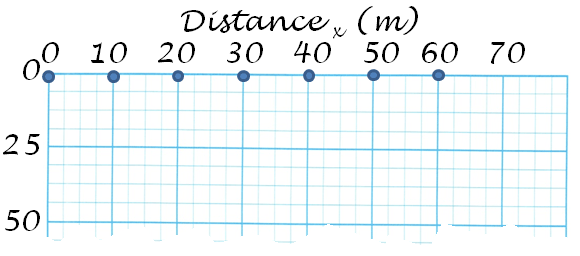Now add the velocity vectors (10m/s for each). The arrows should be 10m long.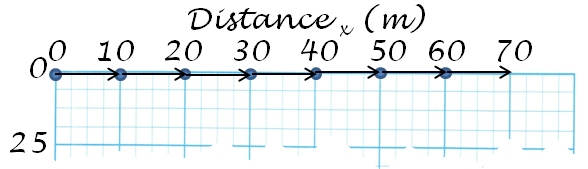A dropped ball will fall straight down with an acceleration of 9.81m/s2.
Using d = ½at2, calculate the total distance the ball has traveled from its
release point for each of the following:

 t(sec) dy(m) [t2x4.905] 0 1 2 3 4 5 6
(Note: the "4.905" is what you get when you multiply ½ x 9.81)
 t(sec) dy(m) [t2x4.905] Answers: 0 0 1 4.9 2 19.6 3 44.1 4 78.5 5 122.6 6 176.6

Now plot this on the graph:

 dx(m) dy(m)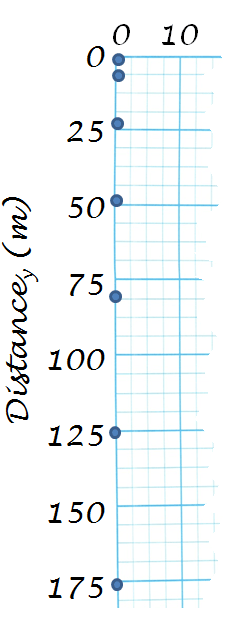0 0 0 4.9 0 19.6 0 44.1 0 78.5 0 122.6 0 176.6

Calculate the velocity of the fall: v = at

 t(sec) v(m/s) [9.81m/s2 x t] 0 0 1 9.8 2 19.6 3 29.4 4 39.2 5 49.1 6 58.9

Now draw in the velocity vectors using the velocities calculated in the table above.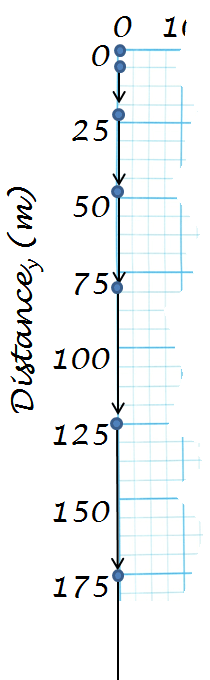Note: the bottom vector goes off the chart which is ok. You get the idea.

Finally, put it all together. Plot the x- and y-coordinates and add the
vertical and horizontal vectors all together.

 dx(m) dy(m) 0 0 10 4.9 20 19.6 30 44.1 40 78.5 50 122.6 60 176.6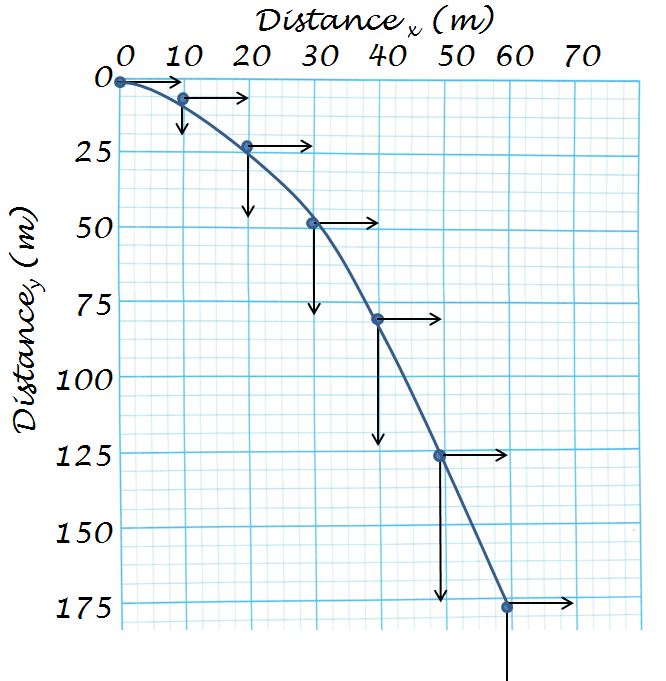With a horizontally launched projectile, it has the properties of a horizontally moving object with constant speed, while behaving like a dropped object accelerating with the pull of gravity. When the projectile is graphed it takes on a curved, parabolic arc, showing the accelerated downward movement while maintaining its constant horizontal speed. As such, when solving horizontal projectile problems, it is usually helpful to divide these two behaviors from each other. Solve all problems as a vertical free-fall problem, then as a horizontal constant motion problem.

To sum up Horizontal Projectile Motion:

• When objects fall, they fall at the acceleration of gravity: 9.81m/s2.
• When objects move horizontally, they continue at the same speed.
• Ignoring air resistance of course!
• A horizontally launched projectile will continue its forward speed (vx)
while falling at an accelerated speed (vy) at 9.81m/s2.
• The time it takes to hit the ground down range will be the same as a
straight drop from the same height.

A virtual demonstration:
TwoBallsGravity.html

Practice Problem:

A plane flying horizontally at an altitude of 490m and having a velocity of 250m/s, drops a supply package to a work crew on the ground. It falls freely without a parachute.

What is the time required to hit the ground?

How far down range will the package travel before hitting the ground?

It helps to draw a simple diagram of the problem: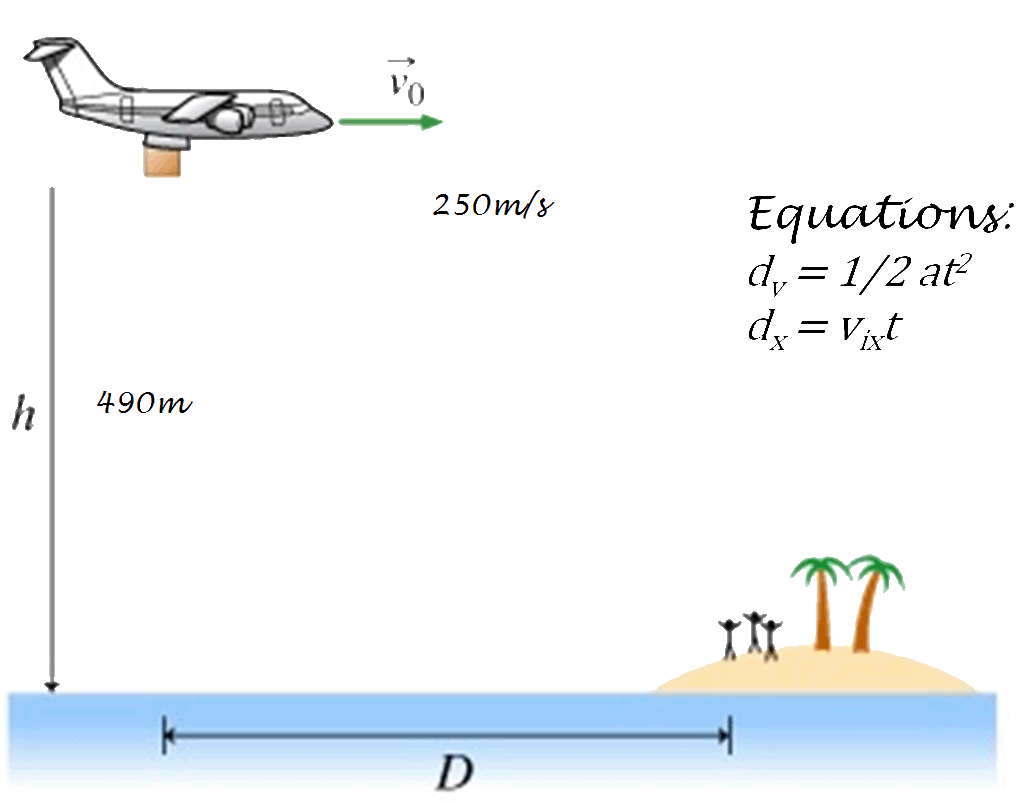### Projectiles at an Angle

How to approach Projectiles at an angle:
Break it into two separate problems: a vertical free-fall problem and a horizontal d = vt problems.

You do this by breaking the initial velocity vector into its x- and y-components. From there it is nothing more than what has been covered earlier.

### Example Problem:

Here is an example of solving a Projectile at an Angle kind of backwards so that you can see how it all comes together- a better understanding of how it works. If you would rather just get to the problems skip to there from here.

### A ball is thrown straight upwards at a velocity of 22.9m/s. How long will it take to reach the top?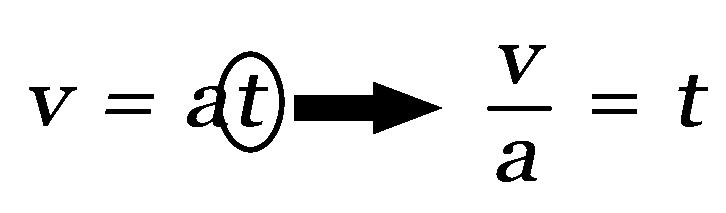Start with the usual v=at equation. Since we need to solve for t we change the equation around to this.

Side note (well, a semi-side note):
Why use v = at instead of the version in the Reference Tables: vf = vi + at?
If you were to use the longer, more formal version you would need to use and keep track of positives (+) and negatives (-).
At which point, for this situation, you would end up having the equation 0 = vi + at. Then you would have to move the vi to the other side of the equal sign to solve for t.

Since one value is zero, you can use the simpler version which actually solves for the trip down. It takes the same amount of time to go up as it does to come down so the math works out to be the same.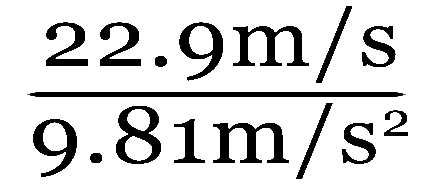Plug in your values and calculate.The t here represents how much time it takes for the ball to reach the top.

### dy = ½at2

The equation we use now is dy = ½at2 (In the original d = vit + ½at2 the vi becomes zero and this term drops out). We don’t use d = v/t because in this situation v is constantly changing.

### dy=(.5)(9.81m/s2)(2.33s)2 =26.6m

Substitute the values and crunch the numbers.

### How far will a ball travel if shot at 32.7m/s horizontally and travels for 2.33s?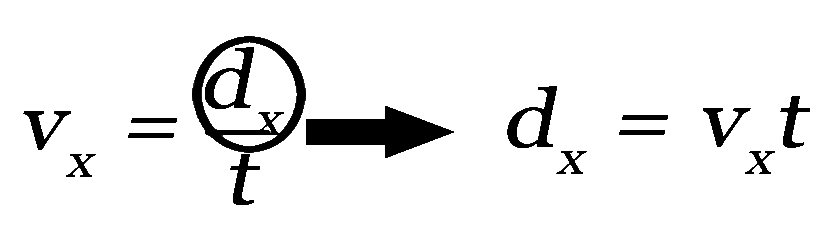We want to find the distance in the x-direction (horizontal) so we start with v = d/t but change it to solve for d: d = vt.

### dx = (32.7m/s)(2.33s) = 76.2m

Substitute the values and crunch the numbers.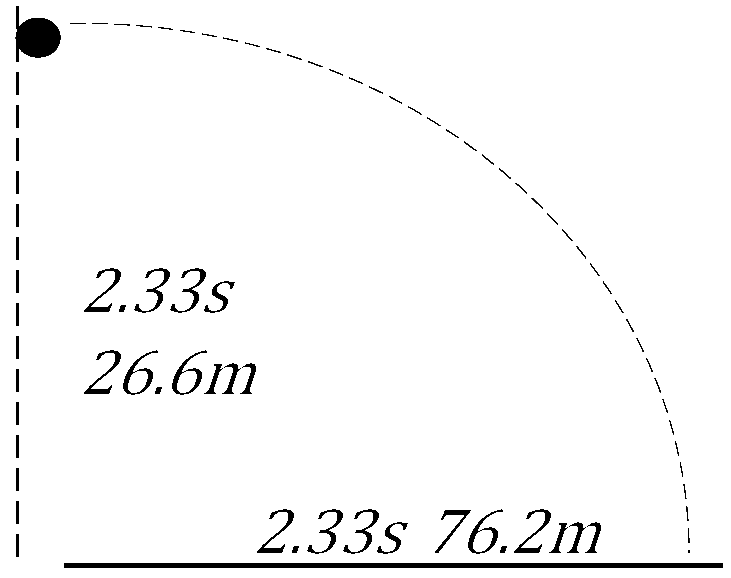Put the vertical and horizontal together and you get a horizontally launched projectile.
It falls down with the acceleration of gravity for 2.33 seconds and travels at constant velocity for the same amount of time to reach76.2m.

### 2.33s up and 2.33s down = 4.66s

The trip up takes the same amount of time it takes for the trip down. The round trip would take 4.66 seconds.

As it is flying up, it travels horizontally for 76.2m. As it falls down it travels another 76.2m for a total horizontal distance of 152.4m.

### What is the angle of launch?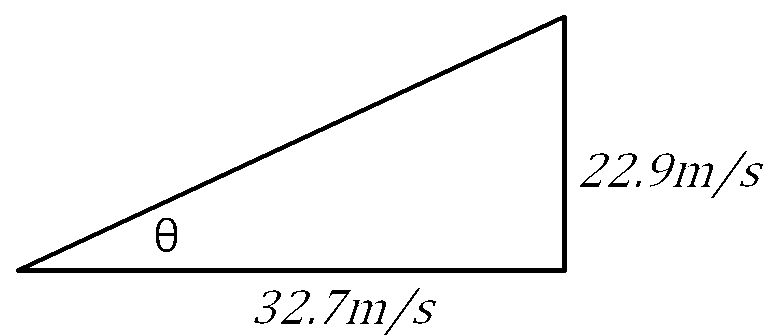Using trig, you can find angle θ by using the tangent- or more specifically: the arctangent (AKA inverse tangent or tan -1). SOH, CAH, TOA⇒ Tangent is opposite over Adjacent.

### tanθ = 22.9⁄32.7

No units needed since they cancel out.

### θ = tan-1(.70)

Rather than getting caught up in the tan-1 use and terminology, tan-1 simply means "what angle has this____ value?"

### θ = 35˚

This is the launch angle.

### What is the velocity of launch?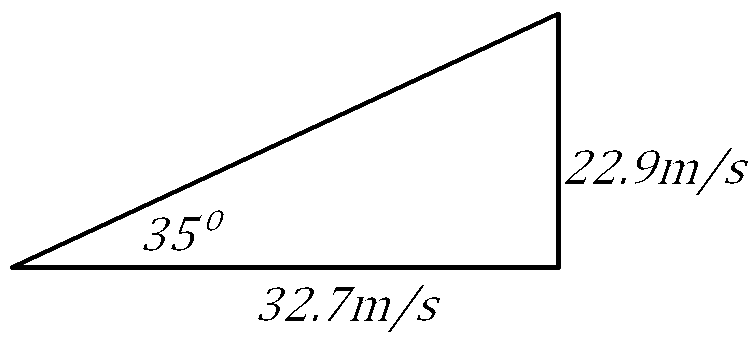### v = 39.9m/s

The velocity of launch is a combination of the vertical and the horizontal speeds. By simply applying the Pythagorean Theorem you can calculate the hypotenuse:
a2 + b2 = c2

## Problem:

### A football player kicks the ball at 25m/s at an angle of 53˚ above the horizontal. (use 10m/s2 for g to make life easy)

• What is the vertical speed?
• horizontal speed?
• time to reach the peak?
• max height of the ball?
• time to peak and land (hang time)?
• total distance traveled (horizontal)?
"The Easy Way"
(Easier only because you don't have to deal with negatives and one term drops out of vf = vi+at)
For the purist
(Keeping all equations and signs in their original form)
What is the vertical speed (vy)?
sin53˚(25m/s) = 20m/s = vy

Same
What is the horizontal speed (vx)?
cos53˚(25m/s) = 15m/s = vx

Same
What is the time to reach the peak (tup)?

vy = at ⇒ t = va

t = (20m/s)/(10m/s2)

t = 2sec

vf = 0m/s at the top

vi = 20m/s initially at the bottom

vf = vi+at

0m/s = 20m/s + (-10m/s2)t
-20m/s -20m/s

-20m/s = -10m/s2 t

t = 2s

What is the max height of the ball (dy)?

dx = 12 at2

dx = (.5)(10m/s2)(2s)(2s)

dx= 20m

Same
What is the time to peak and land (hang time) (tup & down)?

tup + tdown = tupdown

2s + 2s = 4s

vfy = -20 m/s when it hits the grouond

viy = 20m/s initially

vfy = vyiy+at

-20m/s = 20m/s + (-10m/s2)t
-20m/s -20m/s

-40m/s = -10m/s2 t

tupdown = 4s

What is the total distance traveled (horizontal)(dx)?

vx = dt

15m/s = d4s

(15m/s)(4s) = 60m

Same

## Or you can just think of it this way:

Do all of your calculations for horizontal projectiles and double whatever gets doubled:
-time of flight
-distance of flight

This way requires less math skill but you must keep track of what gets doubled, halved and what stays the same.

## For the purist:

The math takes a few more steps but more accurately describes what is happening.

## What angle will give you the furthest shot?

45˚ will give you the best combination of hight and horizontal speed.

Angles that have the same spread from 45˚ (ex: 30˚ & 60˚ are each 15˚ away from 45˚) will land at the same spot. One will take the high road and the other will take the low road. The high road has a longer flight time but a lower horizontal speed while the opposite is true for the low angle.

The video below shows an application of this principle is the MRSI technique used in the military. Multiple Round Simultaneous Impact fires several artillery shells in such a way as to be able to strike the same target at the same time from the same gun. Although, in reality, the gun fires several shots each with different launch velocities (amount of explosive used to fire the shell), it can still be achieved with two shells using the same amount of charge- which is what we are talking about here. The first shell would be fired at 60°, for example, then the gun would be lowered to 30° (45° + and minus 15°) and fired by a timing computer.

## A Classic: The Hunter and the Monkey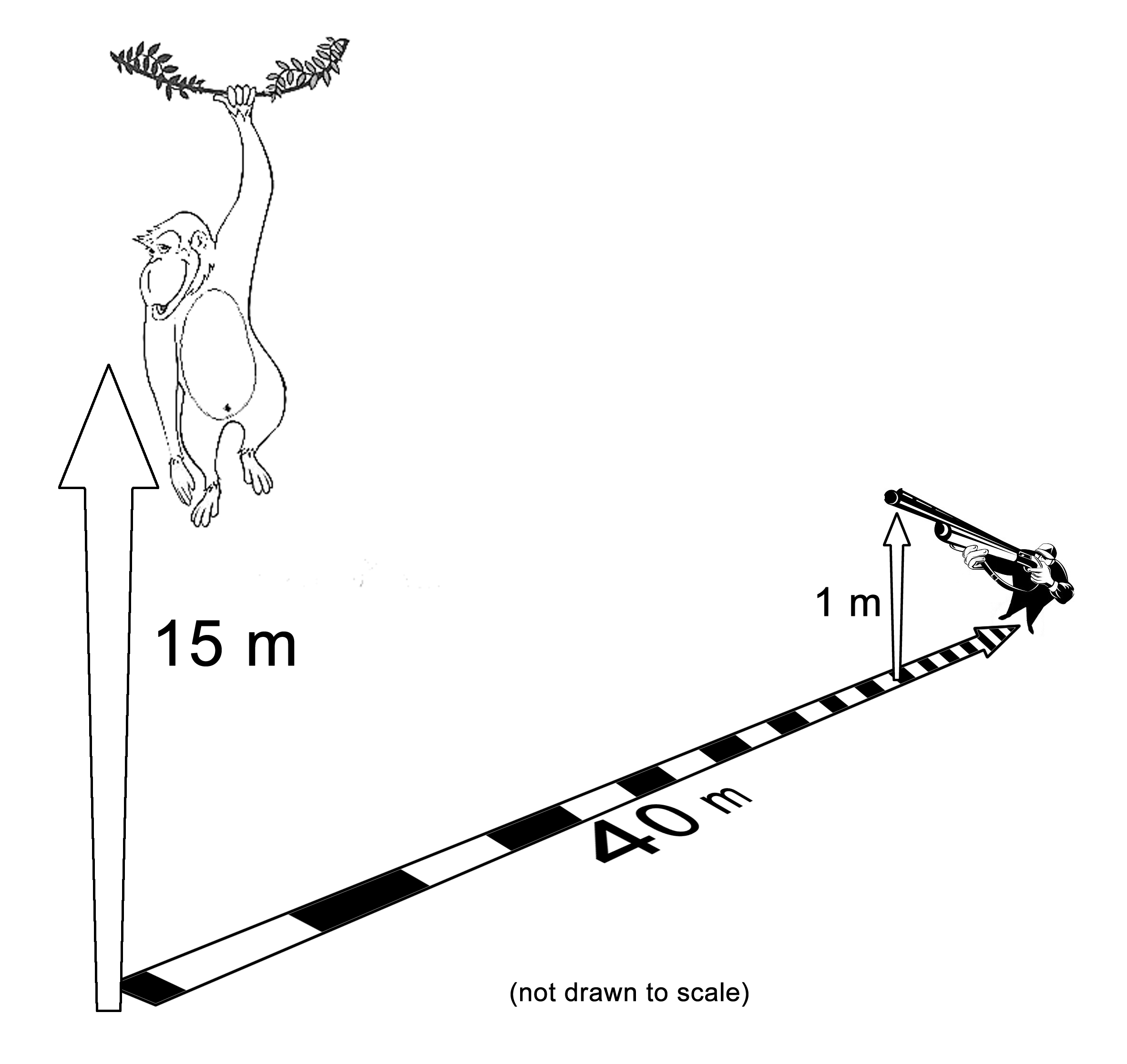The hunter aims his Tippmann A-5 paintball gun directly at the monkey. At the exact moment that the gun fires the monkey lets go of the tree and falls. If the initial velocity of the Tournament Grade Empire Ultra Evil paintball is 30 m/s and the muzzle of the gun is 1 m above the ground.

Will the bullet strike the monkey, pass over his head or go under his feet?

## Do the trig

tanθ = 14m40m

θ = tan-1(.35)

θ = 19.29˚

• The first thing that we need to do is to figure out the angle of the hunter’s gun.
• The tangent of the unknown angle is equal to 14/40.
• Using the inverse tangent function will give us the angle of 19.29˚

vx = (cos19.29˚)(30m/s) = 28.31575m/s

vy = (sin19.29˚)(30m/s) = 9.91049m/s

Find the x- and y-components of the paint ball’s velocity.

Note on sig. figs: This is a mathematical exercise where you can assume that the values are mathematically accurate to an infinite number of decimal places. For the purposes of this exercise, it is necessary to minimize the impact of rounding. As such, I am being excessive with the sig figs.

### How long will it take the paintball to cross the monkey's path as he drops?

d = 40m

vx = dt therefore; t = d⁄vx

40m28.31575m/s = 1.41264 sec

To calculate the time, you take the horizontal distance from the hunter to the monkey and divide it by the horizontal speed.

### Where (vertically) is the paint ball when it reaches the monkey’s path?

dy = vit + 1/2at2

= (9.914049m/s)(1.41264s)
+ ½(-9.81m/s2)(1.41264s)(1.41264s)

= 14.00498m -9.78818m

= 4.2168m above ground

Since we are searching for the vertical position we will use the vertical component of the paintball’s starting speed then factor in the acceleration of gravity downward. (Don’t forget the negative in front of the 9.81)

Note: for this exercise the value of 9.81 was used for gravity. However, if you want to work it out with more precision you can use a value of 9.80665m/s2.

### Where is the monkey when the bullet crosses the path?

dy = vit + ½at2

dy = ½at2

dy = (.5)(-9.81m/s2)(1.41264s)(1.41264s)

= -9.7882m

We use the free-fall equation to see how far the monkey drops in the time we calculated for the paint ball to cross the monkey’s path.
Since the monkey starts falling from rest, his initial velocity is zero and the first term drops out.

The monkey dropped 9.7882m down from the tree.

14m – 9.7882m = 4.2118m
Since the monkey started at 14m above the ground and dropped 9.7882m under that, he was at 4.2118m above the ground at the same time the paint ball crossed his path.

The result?
At the time that the paint ball crosses the Monkey's path - 1.41264 seconds after firing:

The Monkey is 4.2168m above the ground.
The paint ball is 4.2118m above the ground.

Both objects are at the same place at the same time (give or take a little due to rounding issues but close enough to hit the monkey).

# SPLAT!Medina On-Line
Since 1994
Email: mrsciguy@optonline.net